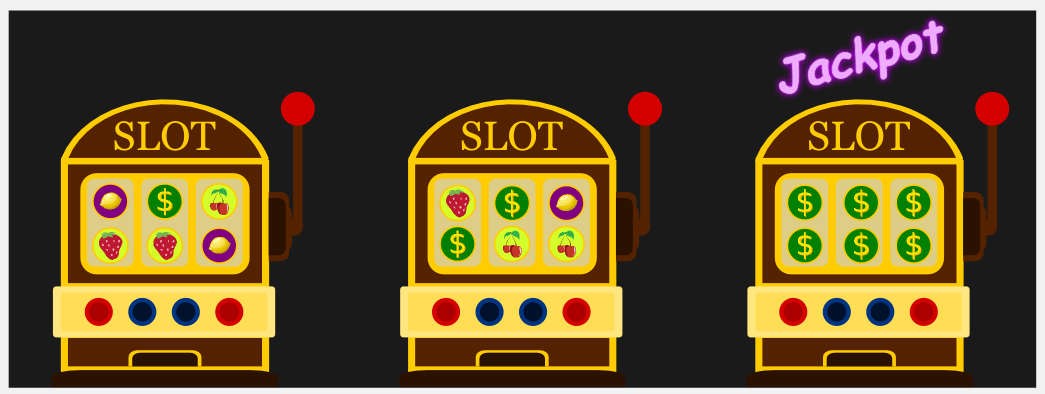# 1.简述

## 问题引入

• 推荐系统冷启动问题常见的一种解决方案就是利用Bandit算法,Bandit算法通常用于解决探索与利用问题,其中解决探索与利用问题的经典案例是MAB问题；• 这里MAB问题有三个概念:臂,收益,遗憾
• 1.臂:实际可以选择的对象,这里的臂可以是推荐系统中的策略或者内容分类,一般情况下臂的数量应该小于选择的次数,这样才能保证收敛。
• 2.收益:选择了臂后对应的单次收益,在推荐系统中可以理解为点击或者购买某个物品
• 3.遗憾:单次收益与最优收益的差
• 目标与建模:
所以我们的目标就是在有限的N次选择中尽量使得累计遗憾最低

$\text{数学建模:} R_{T} = \sum_{i=1}^{T}(W_{opt}-W_{B(i)})\text{,其中}R_T\text{代表累计遗憾;T是总选择次数;} W_{opt}\text{是最优收益;}W_{B(i)}\text{是第i次选择获得的收益}$

$\text{特别地,当每次选择的收益是0或者1时,称为伯努利收益;上式可以化简为如下形式:} R_{T} = T - \sum_{i=1}^{T-N}0\text{,这里的N是取到收益1的次数}$

## UCB算法介绍

### 计算公式

Upper Confidence Bound，即置信区间上界

$score(i) = \frac{N_i}{T} + \sqrt{\frac{2\ln_T}{N_i}} \text{,其中}N_i\text{代表第i个臂收益为1的次数;T是总选择次数。注：这里直接给出了结论,推导部分见附录}$

### 对公式的理解：

• 公式有两部分，加号前面是这个候选臂到目前的平均收益，反应了它的效果，后面的叫做 Bonus，本质上是均值的标准差，反应了候选臂效果的不确定性，就是置信区间的上边界。
• 如果一个臂的选择次数很少，即N很小，那么它的 Bonus 就会较大，在最后排序输出时有优势，这个 Bonus 反映了一个候选的收益置信区间宽度，Bonus 越大，候选的平均收益置信区间越宽，越不确定，越需要更多的选择机会。反之如果平均收益很大，就是说加号左边很大，也会在被选择时有优势。

# 2.实践

import numpy as np

# T个用户/T次曝光
T = 1000
# N个电影/N个电影品类
N = 10

# 保证结果可复现
np.random.seed(888)
# 每部电影累积点击率(理论概率)
true_rewards = np.random.uniform(low=0, high=1, size=N)
# 每部电影当前点击率(实际频率)
estimated_rewards = np.zeros(N)
# 每部电影点击次数
chosen_count = np.zeros(N)
total_reward = 0

def calculate_delta(T, item):
if chosen_count[item] == 0:
return 1
else:
return np.sqrt(2 * np.log(T) / chosen_count[item])

def UCB(t, N):
# UCB得分
upper_bound_probs = [estimated_rewards[item] + calculate_delta(t, item) for item in range(N)]
item = np.argmax(upper_bound_probs)
# 模拟伯努利收益
reward = np.random.binomial(n=1, p=true_rewards[item])
return item, reward

# T个用户/T次曝光依次发生
for t in range(1, T):
# 为第t个用户推荐一部电影，reward = 1 表示用户点击观看，reward = 0 表示用户未点击
item, reward = UCB(t, N)
# print("item, reward = %s,%s" % (item, reward))
# 一共有多少用户接受了推荐/N部电影的点击次数
total_reward += reward

# 更新电影的当前点击率
estimated_rewards[item] = ((t - 1) * estimated_rewards[item] + reward) / t
# print(estimated_rewards[item])
chosen_count[item] += 1
# 输出当前点击率
# print(t,estimated_rewards)
# 输出累积点击率
# print(t,true_rewards)
diff = np.subtract(true_rewards,estimated_rewards)
print(diff)

0.8595606060609418
0.35956060606094176
0.19289393939427513
0.10956060606094176
0.2595606060609418
...
0.01640294418596766
0.016245471958934443
0.01709132465111296
0.01693347823970004
0.016775947837118665


# 3.扩展

## 其他Bandit 算法

• 1.汤普森采样算法
• 2.Epsilon 贪婪算法
• 3.效果对比
完全随机：就是不顾用户反馈的做法。
朴素选择：就是认准一个效果好的，一直推。
Epsilon 贪婪算法：每次以小概率尝试新的，大概率选择效果好的。
UCB：每次都会给予机会较少的候选一些倾向。
汤普森采样：用贝塔分布管理每一个候选的效果。

• 1.新用户:冷启动策略
• 2.新策略/新模型

## 方法扩展

1.LinUCB(加入了特征的UCB)
2.COFIBA算法(将Bandit算法与协同过滤结合使用)

# 4.附录

posted @ 2019-06-20 23:46  混沌战神阿瑞斯  阅读(735)  评论(0编辑  收藏